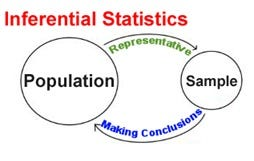# Explain what is meant by the term "inferential statistics.

### October 27, 2019Explain what is meant by the term "inferential statistics
Inferential statistics is one of the two main branches of statistics. Inferential statistics use a random sample of data taken from a population to describe and make inferences about the population. You can measure the diameters of a representative random sample of nails.
What are inferential statistics?
Inferential statistics use a random sample of data taken from a population to describe and make inferences about the population. Inferential statistics are valuable when examination of each member of an entire population is not convenient or possible. For example, to measure the diameter of each nail that is manufactured in a mill is impractical. You can measure the diameters of a representative random sample of nails. You can use the information from the sample to make generalizations about the diameters of all of the nails.Definition: Inferential statistics is a statistical method that deduces from a small but representative sample the characteristics of a bigger population. In other words, it allows the researcher to make assumptions about a wider group, using a smaller portion of that group as a guideline.
What Does Inferential Statistics Mean?
Inferential statistics is one of the two statistical methods employed to analyze data, along with descriptive statistics. The goal of this tool is to provide measurements that can describe the overall population of a research project by studying a smaller sample of it.

This way the researcher can make assumptions about key elements with a fair degree of confidence. Among these inferential tools, we have regression models, normal distributions and R-squared analysis, among others. The study of the smaller sample can be used to explain the variable’s overall behaviour from a whole-population perspective which opens the path for theories and a new hypothesis. In business, inferential statistics are widely used. One of the most common places we can find this method is at forecasting models. These statistical models study a small portion of data to predict the future behaviour of the variables, making inferences based on historical data.FB Page

@ELITE_Mv